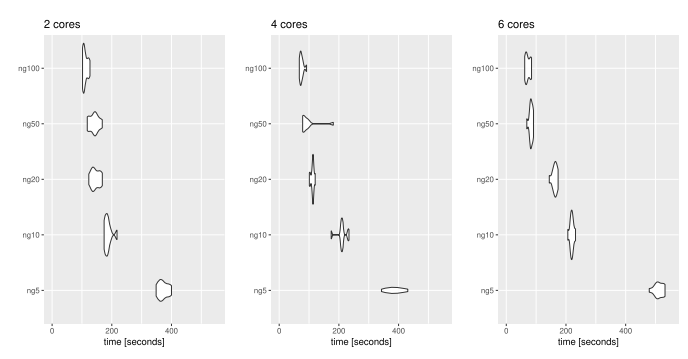Want to share your content on R-bloggers? click here if you have a blog, or here if you don't.

We look at the problem of learning latent structure between two blocks of data through the partial least squares (PLS) approach. These methods include approaches for supervised and unsupervised statistical learning. We review these methods and present approaches to decrease the computation time and scale the method to big data

Given two blocks of data, the PLS approach seeks latent variables which are constructed as linear combinations of the original datasets. These latent variables are constructed according to specific covariance or correlation requirements. As such the latent variables can be used as a data reduction tool that sheds light on the relationship between the datasets. For two blocks of data there are four established PLS methods that can be used to construct these latent variables:

1. PLS-SVD
2. PLS-W2A
3. Canonical correlation analysis (CCA)
4. PLS-R

### Computational speedups

Due to the algorithmic similarity of the different methods some additional computational approaches can be used to speed up the required computation for the PLS approach. In our paper, we consider reducing memory requirements and speeding up computation by making use of the “bigmemory” R package to allocate shared memory and make use of memory-mapped files. Rather than loading the full matrices when computing the matrix cross-product ($$X^TY$$, $$X^TX$$ or $$Y^TY$$) we instead read chucks of the matrices, compute the cross-product on these chucks in parallel, and add these cross-products together, ie. $X^TY = \sum_{c=1}^CX_c^TY_c$ where $$X_c$$ and $$Y_c$$ are matrix chucks formed as the subsets of the rows of $$X$$ and $$Y$$. Additional computational approaches are used for when either p or q are large or when n is very large and data is streaming in while q is small.

### Example on EMNIST

We show an example using PLS regression for a discrimination task, namely the extended MNIST dataset. This data set consists of n = 280,000 handwritten digit images. It contains an equal number of samples for each digit class (0 to 9) where the dimension of the predictors is $$p=784$$ with $$q=10$$ classes. The images are already split into a training set of 240,000 cases and a test set of 40,000 cases. Since we have a large sample size $$n > p, q$$ we opt not to consider regularisation for this example. The PLS-DA method is able to recover an accuracy of 86% in around 3 minutes using 20 latent variables and 2 cores.We investigated the relationship between the number of chunks and the number of cores used in the algorithm. The plot below shows the elapsed computation time for fitting a single component of the PLS discriminant analysis algorithm using 2, 4 or 6 cores (on a laptop equipped with 8 cores). On the vertical axis, $$ngx$$ indicates that $$x$$ chunks were used in our algorithm.